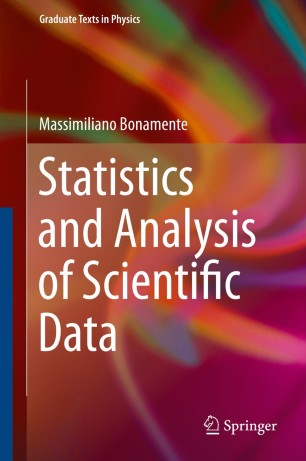# Statistics and Analysis of Scientific Data

• Massimiliano BonamenteTextbook

Part of the Graduate Texts in Physics book series (GTP)

1. Front Matter
Pages i-xv
2. Massimiliano Bonamente
Pages 1-14
3. Massimiliano Bonamente
Pages 15-47
4. Massimiliano Bonamente
Pages 49-75
5. Massimiliano Bonamente
Pages 77-99
6. Massimiliano Bonamente
Pages 101-123
7. Massimiliano Bonamente
Pages 125-141
8. Massimiliano Bonamente
Pages 143-163
9. Massimiliano Bonamente
Pages 165-176
10. Massimiliano Bonamente
Pages 177-187
11. Massimiliano Bonamente
Pages 189-219
12. Back Matter
Pages 221-301

### Introduction

Statistics and Analysis of Scientific Data covers the foundations of probability theory and statistics, and a number of numerical and analytical methods that are essential for the present-day analyst of scientific data. Topics covered include probability theory, distribution functions of statistics, fits to two-dimensional datasheets and parameter estimation, Monte Carlo methods and Markov chains. Equal attention is paid to the theory and its practical application, and results from classic experiments in various fields are used to illustrate the importance of statistics in the analysis of scientific data.

The main pedagogical method is a theory-then-application approach, where emphasis is placed first on a sound understanding of the underlying theory of a topic, which becomes the basis for an efficient and proactive use of the material for practical applications. The level is appropriate for undergraduates and beginning graduate students, and as a reference for the experienced researcher. Basic calculus is used in some of the derivations, and no previous background in probability and statistics is required. The book includes many numerical tables of data, as well as exercises and examples to aid the students' understanding of the topic.

### Keywords

2-variable dataset Monte Carlo Markov chain methods Probability and applied statistics textbook data analysis methods distribution functions of statistics numerical Monte Carlo methods probability textbook random variable theories of probability statistical applications physics statistical data textbook

#### Authors and affiliations

• Massimiliano Bonamente
• 1
1. 1.University of AlabamaHuntsvilleUSA

Industry Sectors